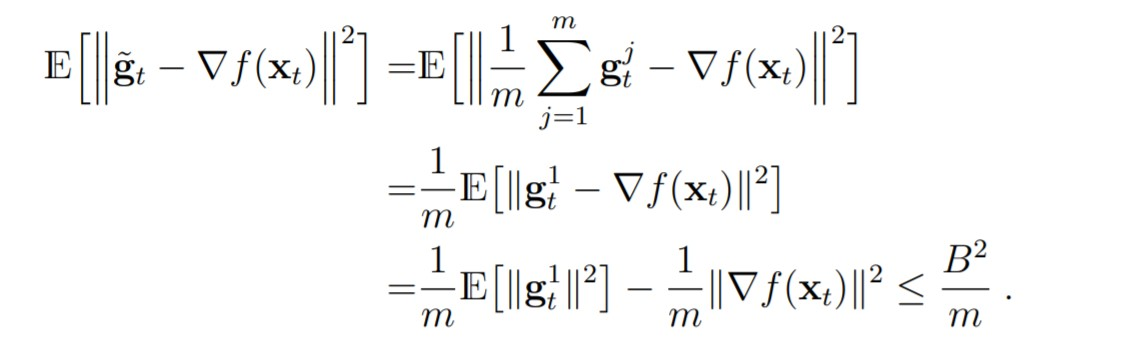### Mini-Batch SGD

Hello, could you explain the second equality? And why the inner product after the third equality is gone?Top comment

The second equality uses the linearity of expectation and summation, and swaps their order.

The third equality use the fact that in expectation the stochastic gradients $$\mathbf{g}_t=\nabla f(\mathbf{x}_t)$$. The cross-terms (inner products) will be equal in expectation to $$-2 \,\text{norm}(\nabla f(\mathbf{x}_t))^2$$.

Hope this helps.

Page 1 of 1